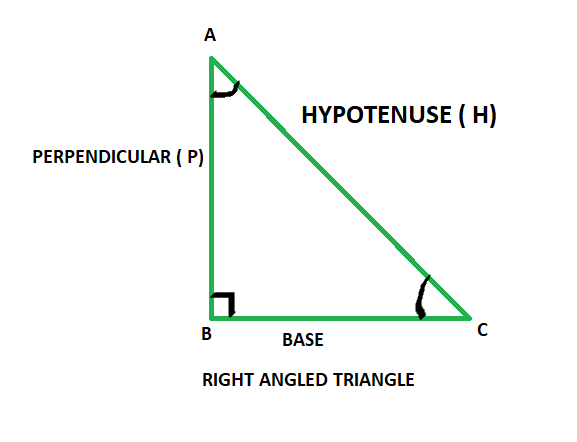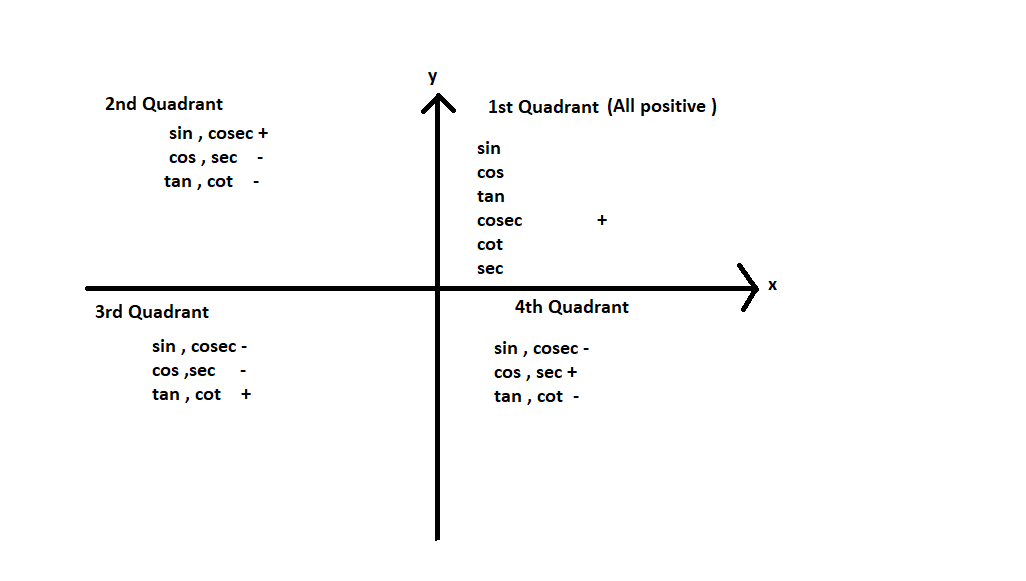# If (1 + cos A)(1 + cos B)(1 + cos C) = (1 – cos A)(1 – cos B)(1 – cos C) then prove that each side is ± sin A sin B sin C

• Last Updated : 10 Dec, 2021

Trigonometry is a discipline of mathematics that studies the relationships between the lengths of the sides and angles of a right-angled triangle. Trigonometric functions, also known as goniometric functions, angle functions, or circular functions, are functions that establish the relationship between an angle to the ratio of two of the sides of a right-angled triangle. The six main trigonometric functions are sine, cosine, tangent, cotangent, secant, or cosecant.

### Trigonometric angles

Angles defined by the ratios of trigonometric functions are known as trigonometry angles. Trigonometric angles represent trigonometric functions. The value of the angle can be anywhere between 0 – 360°.Right angled triangle

As given in the above figure in a right-angled triangle,

• Hypotenuse: The side opposite to the right angle is the hypotenuse, It is the longest side in a right-angled triangle and opposite to the 90° angle.
• Base: The side on which angle C lies is known as the base.
• Perpendicular: It is the side opposite to angle C in consideration.

### Trigonometric Functions

Trigonometry has 6 basic trigonometric functions, they are sine, cosine, tangent, cosecant, secant, and cotangent. Now let’s look into the trigonometric functions. The six trigonometric functions are as follows,

• Sine: It is defined as the ratio of perpendicular and hypotenuse and It is represented as sin θ
• Cosine: It is defined as the ratio of base and hypotenuse and it is represented as cos θ
• Tangent: It is defined as the ratio of sine and cosine of an angle. Thus the definition of tangent comes. out to be the ratio of perpendicular and base and is represented as tan θ
• Cosecant: It is the reciprocal of sin θ and is represented as cosec θ.
• Secant: It is the reciprocal of cos θ and is represented as sec θ.
• Cotangent: It is the reciprocal of tan θ and is represented as cot θ.

According to the above image, Trigonometric Ratios are,

1. Sin θ = Perpendicular / Hypotenuse = AB/AC
2. Cosine θ = Base / Hypotenuse = BC/AC
3. Tangent θ = Perpendicular / Base = AB/BC
4. Cosecant θ = Hypotenuse / Perpendicular = AC/AB
5. Secant θ = Hypotenuse / Base = AC/BC
6. Cotangent θ = Base / Perpendicular = BC/AB

Reciprocal Identities

1. Sin θ = 1/ Cosec θ OR Cosec θ = 1/ Sin θ
2. Cos θ = 1/ Sec θ OR Sec θ = 1 / Cos θ
3. Cot θ = 1 / Tan θ OR Tan θ = 1 / Cot θ
4. Cot θ = Cos θ / Sin θ OR Tan θ = Sin θ / Cos θ
5. Tan θ × Cot θ = 1

Values of Trigonometric Ratios

Identities of Complementary angles are

• sin (90° – θ) = cos θ
• cos (90° – θ) = sin θ
• tan (90° – θ) = cot θ
• cot (90° – θ) = tan θ
• sec (90° – θ) = cosec θ
• cosec (90° – θ) = sec θ

Identities of supplementary angles

• sin (180° – θ) = sin θ
• cos (180° – θ) = – cos θ
• tan (180° – θ) = – tan θ
• cot (180° – θ) = – cot θ
• sec (180° – θ) = – sec θ
• cosec (180° – θ) = – cosec θ### If (1 + cos A)(1 + cos B)(1 + cos C) = (1 – cos A)(1 – cos B)( 1 – cos C) then prove that each side = ± sin A sin B sin C.

Solution:

(1 + cos A)(1 + cos B)(1 + cos C) = (1 – cos A)(1 – cos B)(1 – cos C)

Multiplying both sides of the equation by (1 – cos A)(1 – cos B)(1 – cos C),

[(1 + cos A)(1 – cos A)(1 + cos B)(1 – cos B)(1 + cos C)(1 – cos C)] = [(1 – cos A)²(1 – cos B)²(1 – cos C)²]

[(1 – cos²A)(1 – cos²B)(1 – cos²C)] = [(1 – cos A)(1 – cos B)(1 – cos C)]²

sin² A sin² B sin² C = [(1 – cos A)(1 – cos B)(1 – cos C)]²

[sin A sin B sin C]² = [(1 – cos A)(1 – cos B)(1 – cos C)]²

sin A sin B sin C = (1 – cos A)(1 – cos B)(1 – cos C)

Hence proved

### Similar Problems

Question 1: If sin (A – B) = 1/2, cos (A + B) = 1/2, and 0° < A + B ≤ 90°, A > B, then find the value of A and B.

Solution:

sin(A – B) = 1/2

Sin(A – B) = sin (30° ) ⇢ [sin (30° ) = 1/2]

Equate both the sides,

So, A – B = 30°  ⇢ (1)

And, cos(A + B) = 1/2

⇒ cos(A + B) = cos (60° ) ⇢ [cos (60° ) = 1/2]

By equating both the sides,

A + B = 60°  ⇢ (2)

2A = 90°

⇒ A = 45°

Now, here Putting the value of A in Equation (2),

45° + B =60°

B = 15o

Hence, the value of  A = 45° and B = 15°

Question 2: Evaluate (Sin 45° – Sin 90° + 2Cos 0°) / Tan 30° Tan 60°?

Solution:

Here (Sin 45° – Sin 90° + 2 Cos 0°) / Tan 45° Tan 60°

As per the trigonometric values,

(Sin 45° – Sin 90° + 2 Cos0°) / Tan 45° Tan 60°

= (1/√2 – 1 + 2 × 1) / 1 × √3

= (1/√2 – 1 + 2) / √3

= (1/√2 + 1) / √3

= (1 + √2 / √2) / √3

Question 3: What is the Exact value of cos 270°?

Solution:

Here cos is positive only in 1st and 4th Quadrant.

Therefore, cos(360° – θ) = – cos θ

cos(270°) =  cos(360° – 90°)

cos(270°) = -cos(90°)

cos (270°) = 0 {as per the trigonometry value table}

So the exact value of cos 270° is 0.

My Personal Notes arrow_drop_up